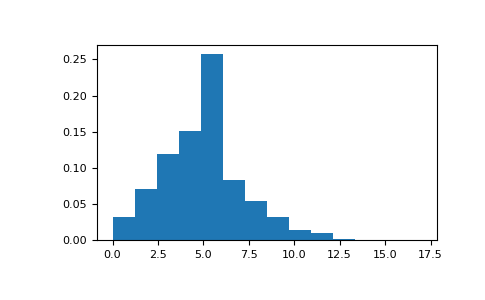#### Previous topic

numpy.random.RandomState.pareto

#### Next topic

numpy.random.RandomState.power

# numpy.random.RandomState.poisson¶

method

RandomState.poisson(lam=1.0, size=None)

Draw samples from a Poisson distribution.

The Poisson distribution is the limit of the binomial distribution for large N.

Note

New code should use the poisson method of a default_rng() instance instead; see random-quick-start.

Parameters
lamfloat or array_like of floats

Expectation of interval, must be >= 0. A sequence of expectation intervals must be broadcastable over the requested size.

sizeint or tuple of ints, optional

Output shape. If the given shape is, e.g., (m, n, k), then m * n * k samples are drawn. If size is None (default), a single value is returned if lam is a scalar. Otherwise, np.array(lam).size samples are drawn.

Returns
outndarray or scalar

Drawn samples from the parameterized Poisson distribution.

Generator.poisson

which should be used for new code.

Notes

The Poisson distributionFor events with an expected separationthe Poisson distributiondescribes the probability ofevents occurring within the observed interval.

Because the output is limited to the range of the C int64 type, a ValueError is raised when lam is within 10 sigma of the maximum representable value.

References

1

Weisstein, Eric W. “Poisson Distribution.” From MathWorld–A Wolfram Web Resource. http://mathworld.wolfram.com/PoissonDistribution.html

2

Wikipedia, “Poisson distribution”, https://en.wikipedia.org/wiki/Poisson_distribution

Examples

Draw samples from the distribution:

>>> import numpy as np
>>> s = np.random.poisson(5, 10000)


Display histogram of the sample:

>>> import matplotlib.pyplot as plt
>>> count, bins, ignored = plt.hist(s, 14, density=True)
>>> plt.show()Draw each 100 values for lambda 100 and 500:

>>> s = np.random.poisson(lam=(100., 500.), size=(100, 2))